• 首先明白梯度下降算法我们能干什么？什么时候可以用到梯度下降？ 我们先进行两个例子，一个二维函数问题，一个三维现实问题： 二维函数：比如我们要求 三维现实问题：你站在一个一个山中，你想要的去谷底，那...
首先要明白梯度下降算法我们能干什么？什么时候可以用到梯度下降？
我们先进行两个例子，一个二维函数问题，一个三维的现实问题：
二维函数：比如我们要求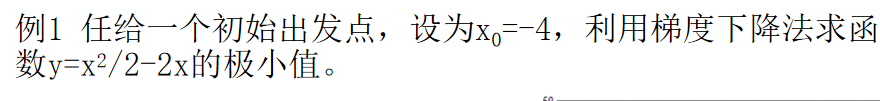三维现实问题：你站在一个一个的山中，你想要的去谷底，那怎么样办才能最快的到达局部谷底呢？要沿着哪个方向进行走动呢？
然后带着问题去思考梯度下降算法。
首先你会有一个函数，比如这个函数有两个参数的函数J(θ_1，θ_2),我们需要一种算法去最小化这个函数
这里我们就需要用到梯度下降算法。
梯度下降算法的步骤：
1.我们开始会有初始数值θ_1，θ_2，一般来说这两个数值为多少没关系，一般可以为0
2.对损失函数求所有 θ 的偏导（梯度）: ∂J(θ)/∂θ_j
3.使用样本数据更新假设函数的不停的同时更新θ_1，θ_2，一定要同时更新，后边会说为什么要同时更更新这个两个变量，更新的参数公式为：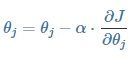（α是为更新步长（调整参数的灵敏度，灵敏度太高容易振荡，灵敏度过低收敛缓慢））
4.直到找到J函数的最小值或者局部最小值比如我们在上图这个点，我想要快速下山，我要怎么办呢？我会根据这个点的变化率最大的方向（也就是偏导数）出发，走一步就再判断一次，最终会走到下图所示的地方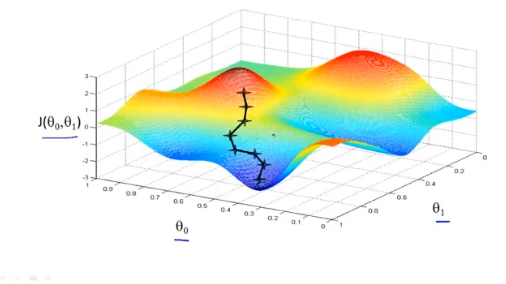但是有一个有趣的事情我们会发现，如果我们开始的位置不同的到结果会不同，正如下图所示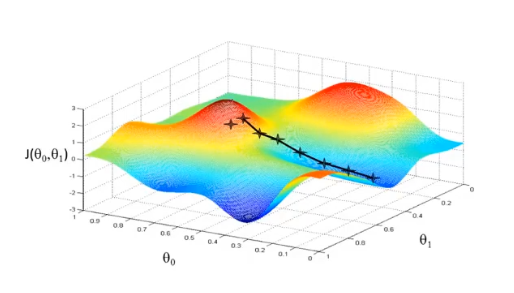这就是梯度下降算法的特点，可以尽快的找到局部最优解或者全体最优解
注意的细节：
我们需要同时更新我们的θ_1，θ_2
算法中应该如下图的步骤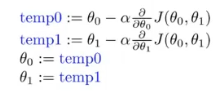而不是这样：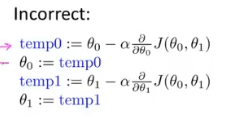然后我们思考更新θ_1，θ_2这两个变量的公式，很明显我们要求最低点的时候，偏导数求导是一个正数，但是我们要向下走因此要加负号，第二个求导是负的，我们本身就是要向下走，因此不用加符号，所有最终结果都是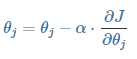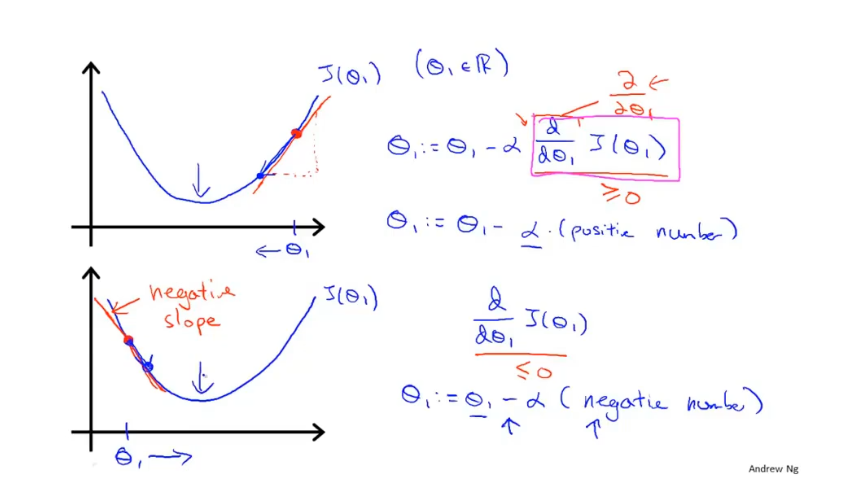然后我们再考虑α这个步长：
α太小：如下图所示，如果太小了 就会导致我们的步数越来越多，我们进行迭代的次数就很大，就会很浪费时间
α太大：如下图所示，如果太大了就会导致我们可能会超过最低点进而无法收敛越来越偏离正确的道路，因此我们需要选定合适的α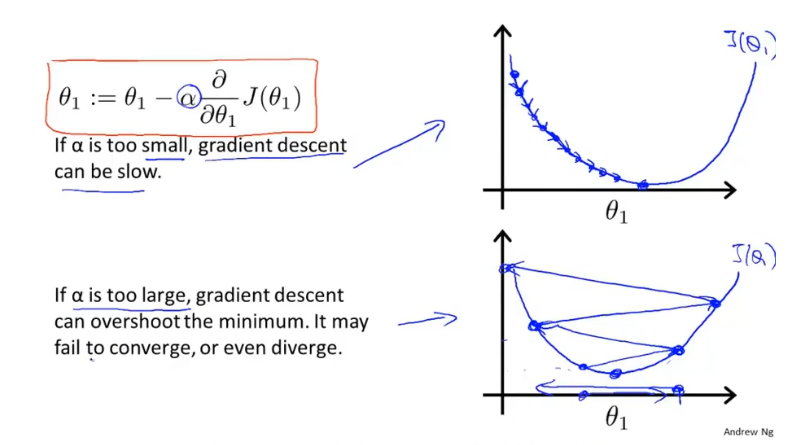大致基本思路就是这样，之后会写一些实际的应用问题
梯度下降算法用于线性回归
批量梯度下降法（BGD）：使用所有样本进行计算，慢但准确度好
首先要导入数据和定义初始的参数以及选择合适的步长，如下图
import numpy as np
import matplotlib.pyplot as plt
import random
spaces = [150, 200, 250, 300, 350, 400, 600]
prices = [6450, 7450, 8450, 9450, 11450, 15450,18450]
#prices=[]
#for i in range(0,len(spaces)):
#prices.append(spaces[i]*prices1[i]/10000)

spaces, prices = np.array(spaces), np.array(prices)

## theta 初始值
theta0 = 0
theta1 = 0

## 如果步长选择不对，则 theta 参数更新结果会不对
step = 0.0000001

#np.ones()生产一个长度跟spaces一样的数组，且数组元素为1，因为theta0为常数
x_i0 = np.ones((len(spaces)))

定义损失函数和theta 0的损失函数和theta 1的损失函数
# 假设函数
def h(x) :
return theta0 + theta1 * x

# 损失函数
def calc_error() :
return np.sum(np.power((h(spaces) - prices),2)) / len(spaces)

# 损失函数偏导数( theta 0)
def calc_delta0() :
return step * np.sum((h(spaces) - prices) * x_i0) / len(spaces)

# 损失函数偏导数( theta 1)
def calc_delta1() :
return step * np.sum((h(spaces) - prices) * spaces) / len(spaces)

利用梯度下降算法进行动态更新
def min1():
return h(spaces)

# 循环更新 theta 值并计算误差，停止条件为
#  1. 误差小于某个值
#  2. 循环次数控制
k = 0
min11 = 100
while True :
delta0 = calc_delta0()
delta1 = calc_delta1()
theta0 = theta0 - delta0
theta1 = theta1 - delta1
plt.figure(1)
plt.scatter(theta0, theta1, c='y')
plt.xlabel('theta0')
plt.ylabel('theta1')
plt.figure(3)
error = calc_error()
plt.scatter(k,error,c='y')
plt.xlabel('k')
plt.ylabel('error')
# print("delta [%f, %f], theta [%f, %f], error %f" % (delta0, delta1, theta0, theta1, error))
k = k + 1
if (k > 500 ) :
break

print(" h(x) = %f + %f * x" % (theta0, theta1))

# 使用假设函数计算出来的价格，用于画拟合曲线
y_out = h(spaces)
plt.figure(2)
plt.scatter(spaces, prices, c='g')
plt.plot(spaces, y_out, c='b')
plt.xlabel('house space')
plt.ylabel('house price')
plt.show()

细节处理：
我们并不是说得到了结果是一条直线就觉得完成了相对应的线性回归，我们需要对损失函数的损失数值进行对比，如果得到如下的图，表示拟合度很高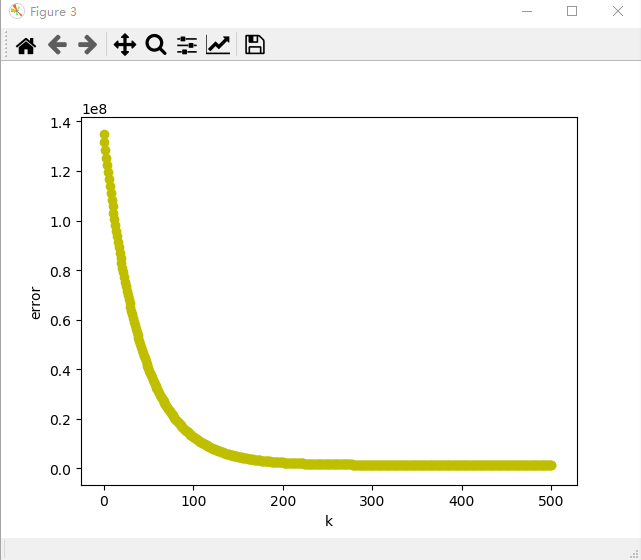在此可以看到损失函数的数值趋近于0代表k只需要200就可以了，当然k越大其误差越小
然后我们可以继续编写随机梯度算法
随机梯度下降法（SGD)：每次使用1个样本进行计算,快但准确度欠缺
然后伪代码如下
## theta 初始值
theta0 = 0
theta1 = 0

## 如果步长选择不对，则 theta 参数更新结果会不对
step = 0.0000015

x_i0 = np.ones((len(spaces)))

# 假设函数

def h(x) :
return theta0 + theta1 * x

# 损失函数
def calc_error() :
return np.sum(np.power((h(spaces) - prices),2)) / len(spaces)

# 损失函数偏导数( theta 0)
def calc_delta0() :
return step * (h(i) - prices[i])

# 损失函数偏导数( theta 1)
def calc_delta1() :
return step * (h(i) - prices[i]) * i

# 循环更新 theta 值并计算误差，停止条件为
#  1. 误差小于某个值
#  2. 循环次数控制
k = 0

while True :
i = random.randint(0,6)
delta0 = calc_delta0()
delta1 = calc_delta1()
theta0 = theta0 - delta0
theta1 = theta1 - delta1
plt.figure(1)
plt.scatter(theta0, theta1,c='g')
plt.xlabel('theta0')
plt.ylabel('theta1')
error = calc_error()
plt.figure(4)
plt.scatter(k,error,c='y')
plt.xlabel('k')
plt.ylabel('error')
# print("delta [%f, %f], theta [%f, %f], error %f" % (delta0, delta1, theta0, theta1, error))
k = k + 1
if (k > 500 ) :
break

print(" h(x) = %f + %f * x" % (theta0, theta1))

# 使用假设函数计算出来的价格，用于画拟合曲线
y_out1 = h(spaces)
plt.figure(2)
plt.plot(spaces, y_out1)
plt.show()

随机梯度算法的核心就是 用单个样本的数值代替整体样本的数值，优点就是速度快但是精度很欠缺，所以需要多k次的运行
我们依旧画出损失函数跟k的关系，如下图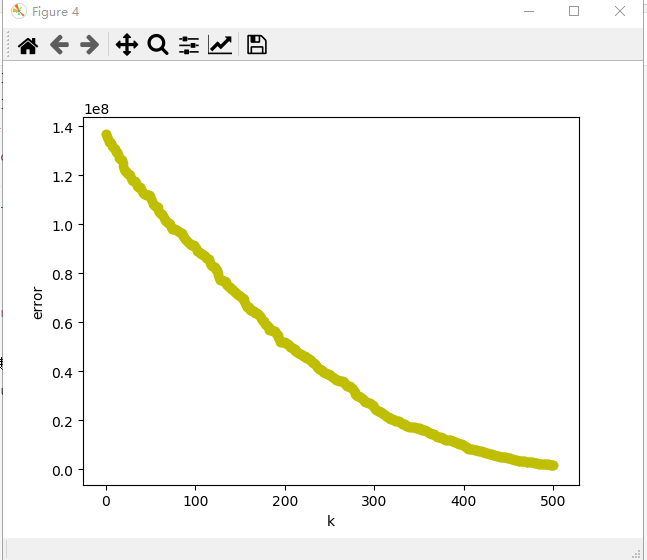我们很明显的可以看出来用批量梯度算法是循环200次，而利用随机梯度算法需要进行500次，但是进行的次数快，每次都是选一个样本进行估计，当然我在这次编程中其步数没有统一，因此其参考价值并没有太大的意义。
最后完整的代码如下
import numpy as np
import matplotlib.pyplot as plt
import random
spaces = [150, 200, 250, 300, 350, 400, 600]
prices = [6450, 7450, 8450, 9450, 11450, 15450,18450]
#prices=[]
#for i in range(0,len(spaces)):
#prices.append(spaces[i]*prices1[i]/10000)

spaces, prices = np.array(spaces), np.array(prices)

## theta 初始值
theta0 = 0
theta1 = 0

## 如果步长选择不对，则 theta 参数更新结果会不对
step = 0.0000001

#np.ones()生产一个长度跟spaces一样的数组，且数组元素为1，因为theta0为常数
x_i0 = np.ones((len(spaces)))

# 假设函数
def h(x) :
return theta0 + theta1 * x

# 损失函数
def calc_error() :
return np.sum(np.power((h(spaces) - prices),2)) / len(spaces)

# 损失函数偏导数( theta 0)
def calc_delta0() :
return step * np.sum((h(spaces) - prices) * x_i0) / len(spaces)

# 损失函数偏导数( theta 1)
def calc_delta1() :
return step * np.sum((h(spaces) - prices) * spaces) / len(spaces)

def min1():
return h(spaces)

# 循环更新 theta 值并计算误差，停止条件为
#  1. 误差小于某个值
#  2. 循环次数控制
k = 0
min11 = 100
while True :
delta0 = calc_delta0()
delta1 = calc_delta1()
theta0 = theta0 - delta0
theta1 = theta1 - delta1
plt.figure(1)
plt.scatter(theta0, theta1, c='y')
plt.xlabel('theta0')
plt.ylabel('theta1')
plt.figure(3)
error = calc_error()
plt.scatter(k,error,c='y')
plt.xlabel('k')
plt.ylabel('error')
# print("delta [%f, %f], theta [%f, %f], error %f" % (delta0, delta1, theta0, theta1, error))
k = k + 1
if (k > 500 ) :
break

print(" h(x) = %f + %f * x" % (theta0, theta1))

# 使用假设函数计算出来的价格，用于画拟合曲线
y_out = h(spaces)
plt.figure(2)
plt.scatter(spaces, prices, c='g')
plt.plot(spaces, y_out, c='b')
plt.xlabel('house space')
plt.ylabel('house price')
plt.show()

## theta 初始值
theta0 = 0
theta1 = 0

## 如果步长选择不对，则 theta 参数更新结果会不对
step = 0.0000015

x_i0 = np.ones((len(spaces)))

# 假设函数

def h(x) :
return theta0 + theta1 * x

# 损失函数
def calc_error() :
return np.sum(np.power((h(spaces) - prices),2)) / len(spaces)

# 损失函数偏导数( theta 0)
def calc_delta0() :
return step * (h(i) - prices[i])

# 损失函数偏导数( theta 1)
def calc_delta1() :
return step * (h(i) - prices[i]) * i

# 循环更新 theta 值并计算误差，停止条件为
#  1. 误差小于某个值
#  2. 循环次数控制
k = 0

while True :
i = random.randint(0,6)
delta0 = calc_delta0()
delta1 = calc_delta1()
theta0 = theta0 - delta0
theta1 = theta1 - delta1
plt.figure(1)
plt.scatter(theta0, theta1,c='g')
plt.xlabel('theta0')
plt.ylabel('theta1')
error = calc_error()
plt.figure(4)
plt.scatter(k,error,c='y')
plt.xlabel('k')
plt.ylabel('error')
# print("delta [%f, %f], theta [%f, %f], error %f" % (delta0, delta1, theta0, theta1, error))
k = k + 1
if (k > 500 ) :
break

print(" h(x) = %f + %f * x" % (theta0, theta1))

# 使用假设函数计算出来的价格，用于画拟合曲线
y_out1 = h(spaces)
plt.figure(2)
plt.plot(spaces, y_out1)
plt.show()

结果分析：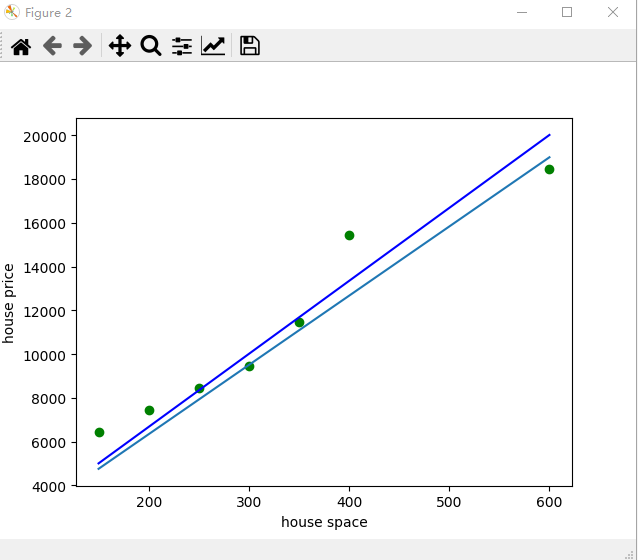蓝色直线是利用批量梯度算法进行的拟合，另一条直线是随机梯度算法进行的拟合
其每次变化的快慢可以通过theta0和theta1的关系进行展示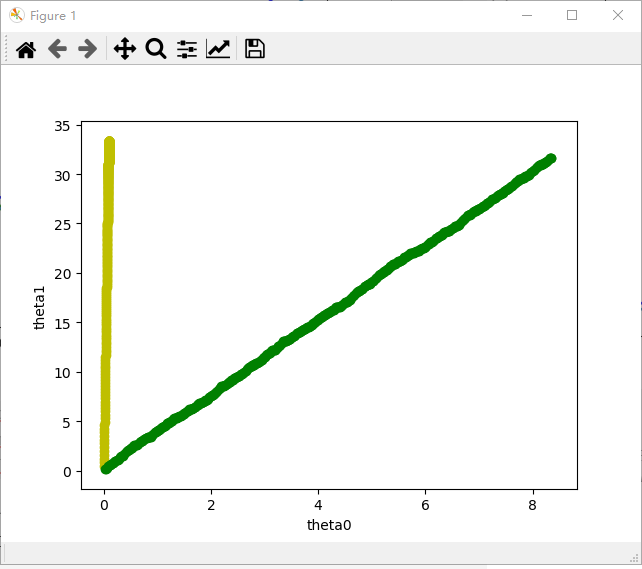当然这仅仅只是参考，对于不同的步数（学习率）会有不同的结果
以下是三种梯度下降算法的特点：
批量梯度下降法（BGD）：使用所有样本进行计算，慢但准确度好
随机梯度下降法（SGD)：每次使用1个样本进行计算,快但准确度欠缺
小批量梯度下降法：每次使用a个样本进行计算，是BGD和SGD的折中方案


展开全文• 梯度下降算法 以下内容参考 微信公众号 AI学习与实践平台 SIGAI 导度和梯度的问题 因为我们做的是多元函数的极值求解问题，所以我们直接讨论多元函数。多元函数的梯度定义为： 其中称为梯度算子，它作用于一个多元...
• 梯度下降和随机梯度下降 目标函数 大多数机器学习或者深度学习算法都涉及某种形式优化。 优化指是改变 以最小化或最大化某个函数 f(x) 任务。 我们通常以最小化 f(x) 指代大多数最优化问题。 最大化可经由最小...
梯度下降和随机梯度下降
目标函数
大多数机器学习或者深度学习算法都涉及某种形式的优化。 优化指的是改变  以最小化或最大化某个函数 f(x) 的任务。 我们通常以最小化 f(x)  指代大多数最优化问题。 最大化可经由最小化算法最小化 -f(x)  来实现。我们把要最小化或最大化的函数称为目标函数或准则。 当我们对其进行最小化时，我们也把它称为代价函数、损失函数或误差函数。 下面，我们假设一个损失函数为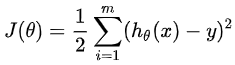其中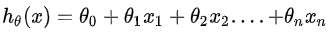然后要使得最小化它。

注意：这里只是假设，不用知道这个目标函数就是平方损失函数等等，然后肯定有人问既然要最小化它，那求个导数，然后使得导数等于0求出不就好了吗？Emmmm…是的，有这样的解法，可以去了解正规方程组求解。说下这里不讲的原因，主要是那样的方式太难求解，然后在高维的时候，可能不可解，但机器学习或深度学习中，很多都是超高维的，所以也一般不用那种方法。总之，梯度下降是另一种优化的不错方式，比直接求导好很多。

梯度下降
我们知道曲面上方向导数的最大值的方向就代表了梯度的方向，因此我们在做梯度下降的时候，应该是沿着梯度的反方向进行权重的更新，可以有效的找到全局的最优解。这个θi的更新过程可以描述为：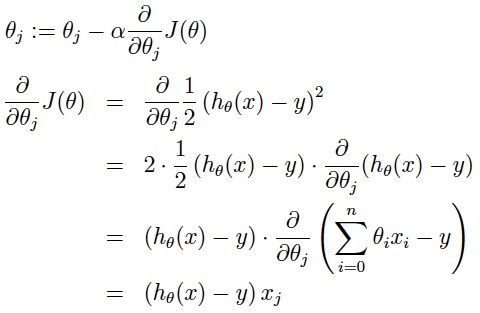[a表示的是步长或者说是学习率（learning rate）]
在直观上，我们可以这样理解，看下图，一开始的时候我们随机站在一个点，把他看成一座山，每一步，我们都以下降最多的路线来下山，那么，在这个过程中我们到达山底（最优点）是最快的，而上面的a，它决定了我们“向下山走”时每一步的大小，过小的话收敛太慢，过大的话可能错过最小值）。这是一种很自然的算法，每一步总是寻找使J下降最“陡”的方向（就像找最快下山的路一样）。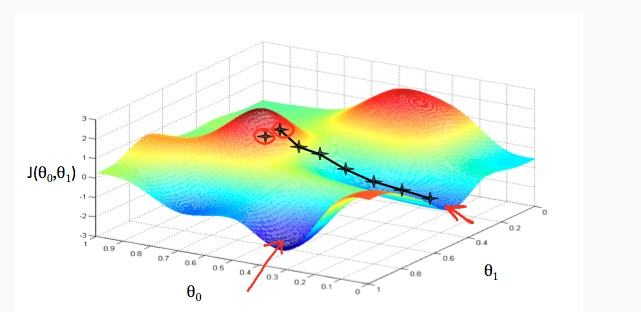当然了，我们直观上理解了之后，接下来肯定是从数学的角度，我们可以这样想，先想在低维的时候，比如二维，我们要找到最小值，其实可以是这样的方法，具体化到1元函数中时，梯度方向首先是沿着曲线的切线的，然后取切线向上增长的方向为梯度方向，2元或者多元函数中，梯度向量为函数值f对每个变量的导数，该向量的方向就是梯度的方向，当然向量的大小也就是梯度的大小。现在假设我们要求函数的最值，采用梯度下降法，结合如图所示：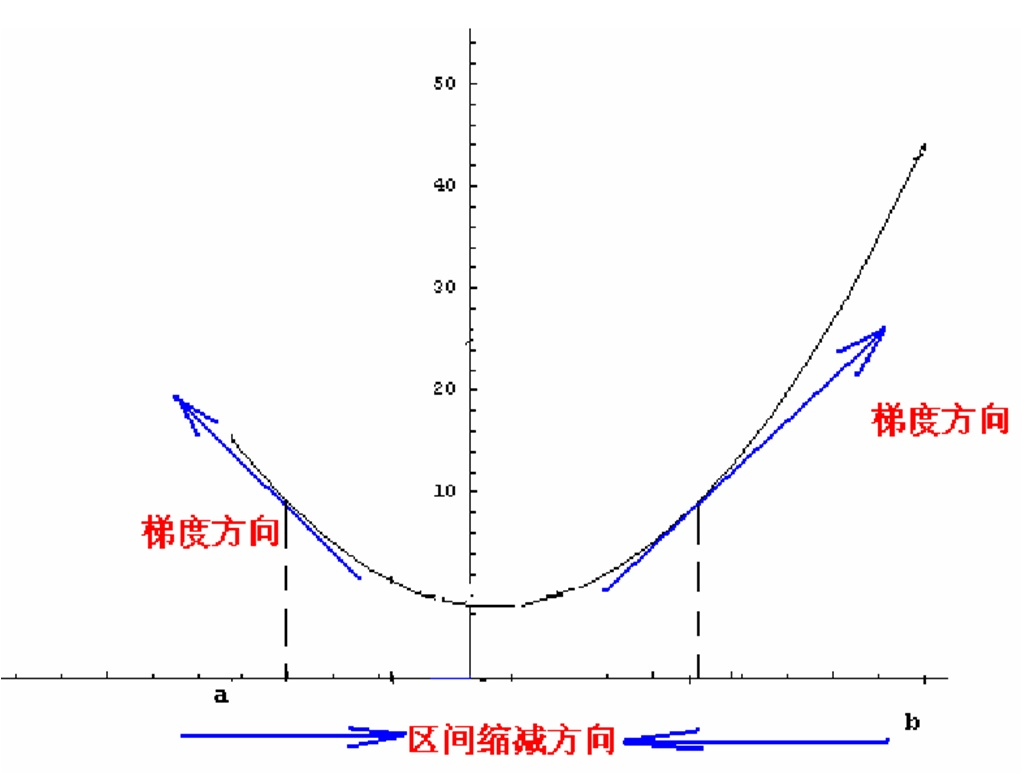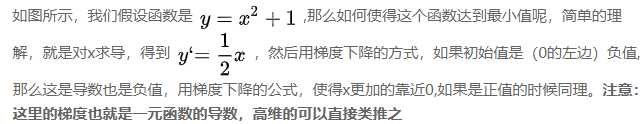注：y’=2x
批量梯度下降
在每次更新时用所有样本，要留意，在梯度下降中，对于θi的更新，所有的样本都有贡献，也就是参与调整θ.其计算得到的是一个标准梯度，对于最优化问题，凸问题，也肯定可以达到一个全局最优。因而理论上来说一次更新的幅度是比较大的。如果样本不多的情况下，当然是这样收敛的速度会更快。但是很多时候，样本很多，更新一次要很久，这样的方法就不合适。下图是其更新公式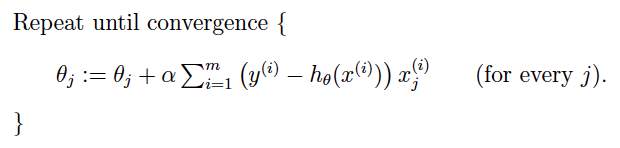或使用：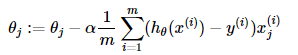随机梯度下降
在每次更新时用1个样本，可以看到多了随机两个字，随机也就是说我们用样本中的一个例子来近似我所有的样本，来调整θ，因而随机梯度下降是会带来一定的问题，因为计算得到的并不是准确的一个梯度，对于最优化问题，凸问题，虽然不是每次迭代得到的损失函数都向着全局最优方向， 但是大的整体的方向是向全局最优解的，最终的结果往往是在全局最优解附近。但是相比于批量梯度，这样的方法更快，更快收敛，虽然不是全局最优，但很多时候是我们可以接受的，所以这个方法用的也比上面的多。下图是其更新公式：，其中m为迭代次数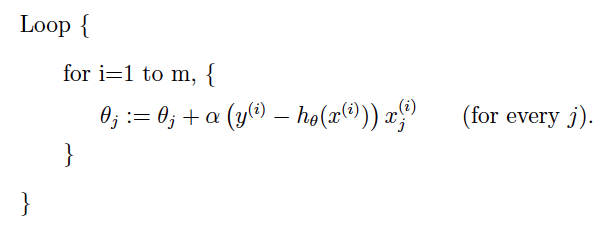或使用：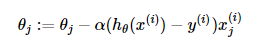mini-batch梯度下降
在每次更新时用b个样本,其实批量的梯度下降就是一种折中的方法，他用了一些小样本来近似全部的，其本质就是只取一个样本可能不够准，用个30个50个样本比随机的要准一些，而且批量还非常可以反映样本的一个分布情况的。在深度学习中，这种方法用的是最多的，因为这个方法收敛也不会很慢，收敛的局部最优也是更多的可以接受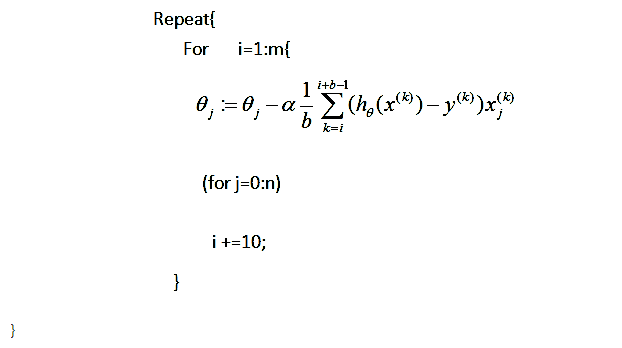具体举例：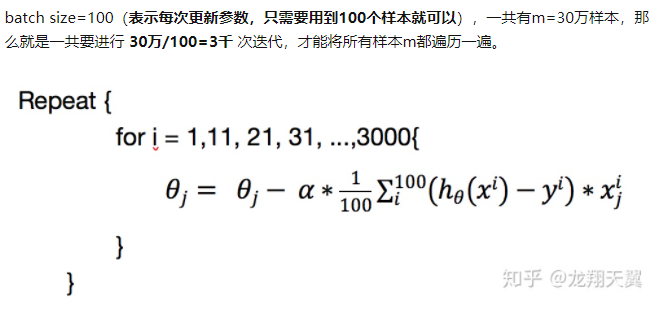收敛
能收敛到的地方：最小值，极小值，鞍点。这些都是能收敛到的地方，也就是梯度为0的点。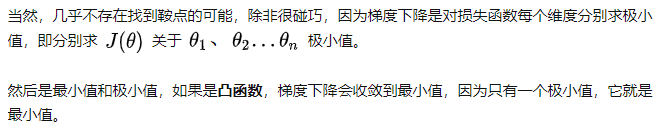总结
随机梯度下降一般来说效率高，收敛到的路线曲折，但一般得到的解是我们能够接受的，在深度学习中，用的比较多的是mini-batch梯度下降。
转载自：https://www.zhihu.com/question/264189719
其他参考网址：
用于回归和分类：https://blog.csdn.net/qq_38150441/article/details/80533891
https://www.cnblogs.com/lliuye/p/9451903.html
https://www.zhihu.com/question/366475349
回归和分类的区别https://www.zhihu.com/question/21329754


展开全文• 我们所说的梯度下降算法一般都默认是批量梯度下降。我们用每个样本去计算每个参数梯度后，将这个梯度进行累加求和 注意这里有一个求和符号，意思就是我们每次计算参数都是用全部样本来计算这个参数变化。 ...
1.批量梯度下降（BGD）
我们所说的梯度下降算法一般都默认是批量梯度下降。我们用每个样本去计算每个参数的梯度后，要将这个梯度进行累加求和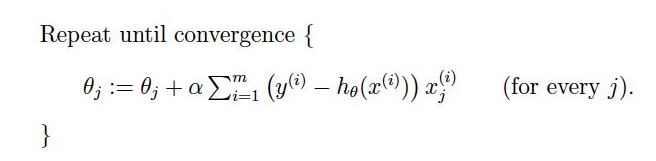注意这里有一个求和符号，意思就是我们每次计算参数都是用全部的样本来计算这个参数的变化。
优点：
1.每次都使用全部全部数据，能更好的代表样本总体，从而更好的指示了正确的梯度下降的方向。
2.对于凸最优化问题，一定能够收敛的全局最优
3 可以并行化
缺点：
每次都使用全部样本进行计算，会导致计算量很大，对于样本数量很大的情况，这种计算会很耗费时间。
2.随机梯度下降(SGD)
与批量梯度下降不同，随机梯度下降每次迭代只使用一个样本来更新参数，使得训练速度更快。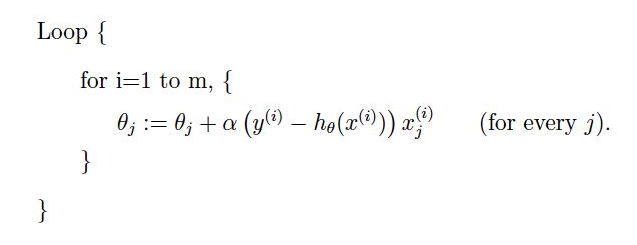注意这里是没有求和符号的，因为只用第i个样本来进行这次更新的计算。
优点：
每次迭代只用一个样本来更新参数，这就使得每次迭代的速度会大大加快，虽然不是每次迭代得到的损失函数都向着全局最优方向， 但是大的整体的方向是向全局最优解的。
缺点：
很大可能会收敛于局部最优
3.小批量梯度下降（MBGD）
小批量梯度下降实际上是在批量梯度下降和随机梯度下降之间做了个中和。
下面我们假设一共1000个样本，每次迭代用10个样本进行参数更新，那么参数的更新如下。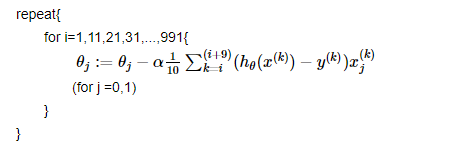对于小批量梯度下降需要注意的就是这个批量batch大小的选择：
如果选择过大：
1.如果batch过大的话，那么我们每个batch就变化很小，就有点接进去批量梯度下降算法
2.内存可能会撑不住
如果选择过小：
会导致其接近于随机梯度下降算法，它的梯度方向可能没那么准确


展开全文• 与之前我有讲过的EM算法类似，梯度下降算法同样是一个优化算法。它所解决的问题是： 求得 θ⋆=arg min⁡L(θ)\theta^{\star}=\argmin L(\theta)θ⋆=argminL(θ) 其中θ\thetaθ是待更新的参数，注意这可以包括...
• 一、为什么提出随机梯度下降算法 注意看梯度下降法权值更新方式（推导过程在上一篇文章中有）  也就是说每次更新权值都需要遍历整个数据集（注意那个求和符号），当数据量小时候，我们还能够接受这种算法，...
• 题目是首先对于一个n维的问题，取初始点为x0 = 0，利用最速下降法，牛顿法，BFGS方法，共轭梯度法求解二次函数的极小点，其中G和b的参数由matlab函数随机生成： , , ; 首先看主函数，这里定义了问题并且实现了...
这段时间学习了优化方法，因为相关的大作业在实现以后我觉得还是很有意思的，所以贴一下分享下，兴许还能帮助到自己的学弟学妹们

题目是首先对于一个n维的问题，取初始点为x0 = 0，利用最速下降法，牛顿法，BFGS方法，共轭梯度法求解二次函数的极小点，其中G和b的参数由matlab函数随机生成：

,

,

;

首先看主函数，这里定义了问题并且实现了题目中的四种方法。具体算法的来源请参照任意一本优化方法教科书，我这里只说工程上的实现。

但是要注意这里面有两个自定义的函数searchReg和bisection，searchReg的功能是得到在做精确线搜索之前所需要的搜索区间;bisection函数的功能是用精确线搜索方法找到迭代步长。

%main函数
clear
n = 282;
x0 = zeros(n,1);
a = unidrnd(10,n,1);
G = a * a'+ unidrnd(2) * eye(n);
b = 0.5 * G * ones(n,1);
f = @(x)1/2 * x'* G * x - b' * x;
deltaf = @(x)G * x - b;
epsilon = 0.1;
%使用最速下降法，并开始计时
tic
x_steepDes = x0;
deltaf_x = deltaf(x_steepDes);
while norm(deltaf_x,2) > epsilon
[lamda1,lamda2,t0] = searchReg(f,deltaf(x_steepDes),x_steepDes);%得到线搜索初始区间
lamda_speed = bisection(lamda1,lamda2,f,deltaf(x_steepDes),x_steepDes);%得到步长
x_steepDes = x_steepDes - lamda_speed * deltaf_x;
deltaf_x = deltaf(x_steepDes);
end
%输出此时得到的最优值
fprintf('使用最速下降方法得到的最小值为: %e\n',f(x_steepDes));
toc
tic
%使用牛顿法，可以一次得到最小值
x_newton = x0 - inv(G) * deltaf(x0);
fprintf('使用牛顿方法得到的最小值为: %e\n',f(x_newton));
toc
tic
%使用共轭梯度法，最多进行 n=282 次迭代
x_conjugateGra = x0;
x_cg = x_conjugateGra;
BREAK = false;
while true
g0 = deltaf(x_conjugateGra);s0 = -g0;
k = 0;
while k < 282
[lamda1,lamda2,t0] = searchReg(f,-s0,x_cg);
lamda_cg = bisection(lamda1,lamda2,f,-s0,x_cg);
x_cg = x_cg + lamda_cg * s0;
g1 = deltaf(x_cg);
if norm(g1,2) < epsilon
BREAK = true;
break;
end
mu = (norm(g1,2)^2)/(norm(g0,2)^2);
s1 = -g1 + mu * s0;
k = k + 1;
g0 = g1;
s0 = s1;
end
if(BREAK == true)
break;
end
x_conjugateGra = x_cg;
end
fprintf('使用共轭梯度方法得到的最小值为: %e\n',f(x_cg));
toc
tic
% 使用BFGS方法，初始H = I
H0 = eye(n);
x_BFGS0 = x0;
while norm(deltaf(x_BFGS0),2) > epsilon
[lamda1,lamda2,t0] = searchReg(f,H0 * deltaf(x_BFGS0),x_BFGS0);
lamda_BFGS = bisection(lamda1,lamda2,f,H0 * deltaf(x_BFGS0),x_BFGS0);
delta_x = lamda_BFGS * H0 * deltaf(x_BFGS0);
x_BFGS1 = x_BFGS0 - delta_x;
delta_g = G * delta_x;
mu_k = 1 + (delta_g' * H0 * delta_g) / (delta_x' * delta_g);
H1 = H0 + (mu_k * (delta_x * delta_x') - H0 * delta_g * delta_x' - delta_x * delta_g' * H0) ...
/ (delta_x' * delta_g);
x_BFGS0 = x_BFGS1;
H0 = H1;
end
fprintf('使用BFGS方法得到的最小值为: %e\n',f(x_BFGS0));
toc

searchReg函数代码为：

%searchReg
function [lamda1,lamda2,t0] = searchReg(f,current_df,current_x)
%   该函数返回搜索区间和初始点，输入参数为函数f和当前搜索方向current_df，以及当前位置x
t0 = 0.3;
h = 0.1;
f0 = f(current_x - t0 * current_df);
t2 = t0 + h;
f2 = f(current_x - t2 * current_df);
if f2 <= f0
t1 = t0 + h;
out = domain(t0,t1,t2,h,f,current_df,current_x);
lamda1 = out(1);
lamda2 = out(2);
t0 = out(3);
else
h = -h;
t1 = t0 + h;
out = domain(t0,t1,t2,h,f,current_df,current_x);
lamda1 = out(1);
lamda2 = out(2);
t0 = out(3);
end
end

事实上该得到搜索区间的方法也是通过迭代得到的，而它又很容易通过递归实现（具体看优化方法书：得到初始搜索区间的方法）所以这里又利用了另外一个函数domain来重复这一递归的过程。

%domain
function [ret] = domain(t0,t1,t2,h,f,current_df,current_x)
%利用递归实现函数截止
if f(current_x - t1 * current_df) > f(current_x - t0 * current_df)
ret = [min(t1,t2),max(t1,t2),(t1 + t2)/2];
else
h = 2 * h;
t2 = t0;
t0 = t1;
t1 = t0 + h;
ret = domain(t0,t1,t2,h,f,current_df,current_x);
end
end

而bisesction就比较简单了，这里利用二分法来查找最佳的行进步长。递归仍然是算法最简单的实现方式。。

%bisection
function [t0] = bisection(t1,t2,f,current_df,current_x)
%二分法做精确线搜索
epsilon0 = 0.000001;
if abs(t1 - t2) < epsilon0
t0 = (t1 + t2 )/2;
else
phi1 = f(current_x - t1 * current_df);
phi2 = f(current_x - t2 * current_df);
if phi1 < phi2
t0 = bisection(t1,(t1 + t2)/2,f,current_df,current_x);
else
t0 = bisection((t1 + t2)/2,t2,f,current_df,current_x);
end
end

end

运行主函数就自动往下执行并调用相关的函数。而控制台的输出显示为：

>> main
使用最速下降方法得到的最小值为: -2.881110e+05
历时 0.989185 秒。
使用牛顿方法得到的最小值为: -2.881110e+05
历时 0.003716 秒。
使用共轭梯度方法得到的最小值为: -2.881110e+05
历时 0.009172 秒。
使用BFGS方法得到的最小值为: -2.881110e+05
历时 0.012209 秒。

多次执行以后我们是可以发现最速下降法速度最慢，其他方法对多数情形有较好的速度。这一结论与教科书中的结论是一致的。

最后说说最近很火的机器学习和深度学习相关的，当要优化的问题是一个更复杂且更接近实际的问题时，它就可能没有二次函数这么好的性质了。机器学习中有一个词叫做学习率，其实就是我们做线搜索所得到的步长。只不过在机器学习中至少在机器学习的入门阶段利用的是固定步长，设置一个比较小的值可以更精确的得到极小值。但是可能计算速度就会比较慢。


展开全文• 梯度下降算法相当于就是去求解一个函数。 回归问题 我们再求解一个函数极值时候一般都是求他导数，然后找出导数值为0时x取值。 梯度下降跟这个也很类似，就是找出损失函数极小值。而找出他极小值...机器学习 人工智能 深度学习 python
• 这边有个问题，为什么Sigmoid和tanh激活函数，z很大或很小时候，也就是函数斜率/梯度很小接近于0时候，会拖慢梯度下降算法？ 我自己理解是：z无限大时候，梯度变化会很慢，所以在反向传播求导过程中，梯度...深度学习 人工智能
• ## 理解神经网络中的Dropout

万次阅读 多人点赞 2017-09-28 10:32:06
注意是暂时，对于随机梯度下降来说，由于是随机丢弃，故而每一个mini-batch都在训练不同网络。过拟合是深度神经网（DNN）中一个常见问题：模型只学会在训练集上分类，这些年提出许多过拟合问题的解决方案，...神经网络 深度学习
• ## GBDT理解二三事

万次阅读 多人点赞 2015-02-10 16:59:44
一、理解GBDT当然从GB(Gradient Boosting)和DT(Decision Tree)两个角度来...注意，这里的梯度下降法是在函数空间中通过梯度下降法寻找使得LOSS最小一个函数，区别于传统的梯度下降法选择一个方向；那么问题就来Gbdt
• 第一节内容：包括神经网络的基础知识，BP网络的特点，bp主要应用的场合，使用时应注意的问题。 什么是神经网络？ 神经网络是由很多神经元组成的，首先我们看一下，什么是神经元 ...matlab
• 最先讲到梯度下降算法。 首先设定初始值theta0，thata1. 其次，重复迭代直到收敛{ 注意要同时更新theta0，theta1. } alpha表示学习速率。代表着下降速度。 这一种方法最大问题是：如何辨别求解结果是局部...机器学习
• 作为一枚机器学习的爱好者，逻辑回归算是一个简单入门的算法，原理比较简单，但是自己手动实现逻辑回归有一些要注意的事项： 第一是步长选择的问题，根据你的数据大小来选择。 第二是自己手动可选择加不加入常数项，...
• 然后转化为最优化问题，用梯度下降等方法进行更新 最后模型收敛，得到我们模型参数 注意在找损失函数时有时会遇到这样情况： 找到损失函数对该模型参数并不是连续可导，无法进行更新。这样话我们就要...机器学习
• 7.1 过拟合的问题 7.2 代价函数 7.3 正则化线性回归 7.4 正则化的逻辑回归模型 第四周 第八、神经网络：表述(Neural Networks: Representation) 8.1 非线性假设 8.2 神经元和大脑 8.3 模型表示1 8.4 模型表示2 8.5...coursera
• 2、梯度下降算法 3、均值归一化 4、最终运行结果 5、使用scikit-learn库中线性模型实现 二、逻辑回归 1、代价函数 2、梯度 3、正则化 4、S型函数（即） 5、映射为多项式 6、使用优化方法 7、运行结果 8、...
• 2.9 逻辑回归的梯度下降（Logistic Regression Gradient Descent） 2.10 梯度下降的例子(Gradient Descent on m Examples) 2.11 向量化(Vectorization) 2.12 更多向量化例子（More Examples of Vectorization） ...
• 随机梯度下降算法 中文资料： 机器学习中数学基本知识 统计学习方法 大学数学课本（从故纸堆里翻出来^_^） 编程工具 斯坦福资料： Python复习 TensorFlow教程 中文资料： 廖雪峰python3教程 莫烦...